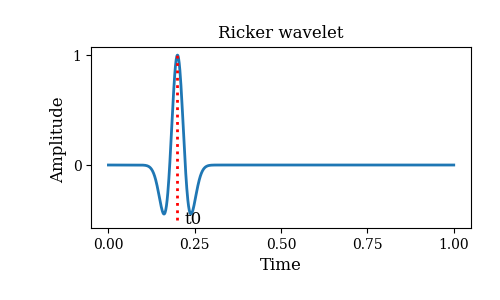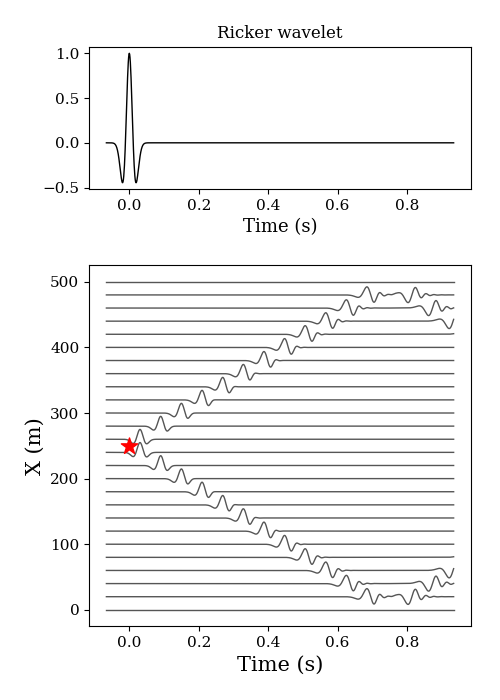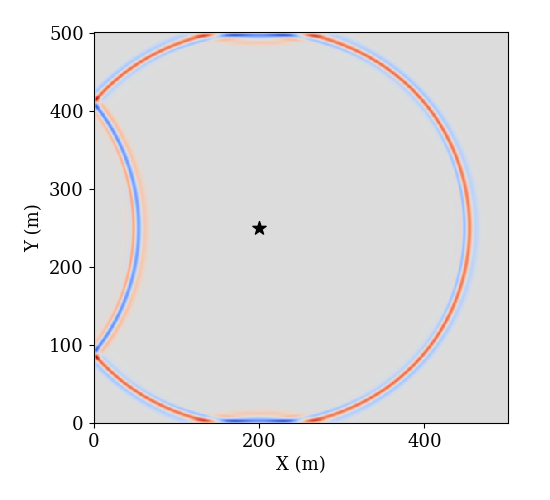## 1 Acoustic wave equation

The acoustic wave equation in vector form is given by

$$p_{tt}=c^2 \nabla^2 p \tag{1}$$

where, $p$ is the acoustic pressure, $c$ is the propagation speed of acoustic wave, $p_{tt}$ is the second time derivative of $p$, and $\nabla^2$ denotes the $Laplacian$ operator. We will discretize the equation using finite difference method in the following parts.

## 2 Propagation in 1D space

The Taylar’s series of $p(t+\Delta t)$ and $p(t-\Delta t)$ are given by

\left \{ \begin{aligned} p(t+\Delta t) &= p(t) + p’(t)\Delta t + \frac{1}{2}p’'(t)\Delta t^2 + \mathcal{O}(\Delta t^2)\\ p(t-\Delta t) &= p(t) - p’(t)\Delta t + \frac{1}{2}p’'(t)\Delta t^2 + \mathcal{O}(\Delta t^2) \end{aligned} \right. \tag{2},

so we get $p_{tt}$ in discrete form as

$$p_{tt}=\frac{\partial ^2 p(x, t)}{\partial t^2}= \frac{p(x, t+\Delta t)-2p(x, t)+p(x, t-\Delta t)}{\Delta t^2} \tag{3}.$$

Similarly, the discretae form of $\nabla^2p$ becomes

$$\nabla^2 p = \frac{p(x+\Delta x)-2p(x)+p(x-\Delta x)}{\Delta x^2} \tag{4}.$$

Lastly, we get the 3-point finite difference in time and space domains of 1D acoustic wave and the updated pressure is given by

\begin{aligned} p(x, t+\Delta t) &= 2p(x, t) - p(x, t-\Delta t)\\ &+ \frac{c^2\Delta t^2}{\Delta x^2}[p(x+\Delta x, t)-2p(x, t)+p(x-\Delta x, t)] \end{aligned} \tag{5}.

For simplicity, we rewrite eq. (5) as $$p^{i+1}_j = 2p^i_j-p^{i-1}_j + \frac{c^2\Delta t^2}{\Delta x^2}[p^i_{j+1}-2p^i_j+p^i_{j-1}] \tag{6},$$

where, $i, j$ indicate the indices of time and space, respectively.

## 3 Propagation in 2D space

For the acoustic wave in 2D homogenous space domains, we have

$$\frac{\partial ^2p(x, t)}{\partial t^2} = c^2 [\frac{\partial ^2p(x, t)}{\partial x^2} + \frac{\partial ^2p(x, t)}{\partial y^2}] \tag{7}.$$

Here, we use $i, j, k$ to indicate the indices of time, $x$ and $y$, respectively. Thus, $$p^{i+1}_{j, k}=2p^i_{i, j}-p^{i-1}_{j,k}+c^2\Delta t^2 [\frac{p^i_{j, k+1}-2p^i_{j,k}+p^i_{j,k-1}}{\Delta x^2}+ \frac{p^i_{j+1, k}-2p^i_{j,k}+p^i_{j-1,k}}{\Delta y^2}+ ] \tag{8}.$$

## 4 Stability condition

$$\frac{c \Delta t}{\Delta x} \le 1 \tag{9}.$$

## 5 Numerical examples

We use Ricker wavelet as the excitation of the acoustic wave equation, and the Ricker wavelet is given by $$r(t)=\{1-2[\pi f_0 (t-t_0)]^2\} e^{-[\pi f_0 (t-t_0)]^2} \tag{10},$$ where, $f_0$ is the center frequency, and $t_0$ is the shift time. The following figure shows the shape of Ricker wavelet waveform in time domain with given parameters.### 5.1 1D

  1 2 3 4 5 6 7 8 9 10 11 12 13 14 15 16 17 18 19 20 21 22 23 24 25 26 27 28 29 30 31 32 33 34 35 36 37 38 39 40 41 42 43 44 45 46 47 48 49 50 51 52  import numpy as np import matplotlib.pyplot as plt def aco_1d(nt, dt, nx, dx, sx, c, s): # Initilization of pressure matrix u = np.zeros((nt, nx)) A = (c*dt/dx) ** 2 for i in range(1, nt-1): for j in range(1, nx-1): # Set value of pressure as source excitation if j == sx: u[i+1, j] = s[i] # Update pressure else: u[i+1, j] = 2*u[i, j] - u[i-1, j] \ + A*(u[i, j+1]-2*u[i, j]+u[i, j-1]) return u def main(): # No. of time, time inverval and acoustic wave speed nt = 1001; dt = 1e-3; c = 340 # Center frequency and shift time of Ricker wavelet f0 = 20; t = np.arange(nt) * dt; t0 = nt//15*dt s = (1-2*(np.pi*f0*(t-t0))**2) * np.exp(-(np.pi*f0*(t-t0))**2) t -= t0 # No. of space nx = 501; dx = 1 # Position index of source sx = nx // 2 x = np.arange(nx) * dx u = aco_1d(nt, dt, nx, dx, sx, c, s) plt.figure(figsize=(5, 7)) ax1 = plt.subplot2grid((3, 1), (0, 0), rowspan=1, colspan=1) ax1.plot(t, s, lw=1, color='k') ax1.set_xlabel('Time (s)', fontsize=13) ax1.set_title('Ricker wavelet', fontsize=12) ax1.tick_params(labelsize=11) ax2 = plt.subplot2grid((3, 1), (1, 0), rowspan=2, colspan=1) for i in range(0, len(u), 20): ax2.plot(t, u[:, i]*15+i, lw=1, color='#555555', zorder=0) ax2.scatter(0, sx*dx, marker='*', s=150, facecolor='r', zorder=1) ax2.set_xlabel('Time (s)', fontsize=13) ax2.set_ylabel('X (m)', fontsize=15) ax2.set_xlabel('Time (s)', fontsize=15) ax2.tick_params(labelsize=11) plt.tight_layout() plt.show() if __name__ == '__main__': main()### 5.2 2D

  1 2 3 4 5 6 7 8 9 10 11 12 13 14 15 16 17 18 19 20 21 22 23 24 25 26 27 28 29 30 31 32 33 34 35 36 37 38 39 40 41 42 43 44 45 46 47 48 49 50  import numpy as np import matplotlib.pyplot as plt #from numba import jit #@jit(nopython=True) def main(): nx = 501 ny = 501 dx = 0.5 nt = 401 dt = 1e-3 c = 340 rx = 150 ry = 150 sx = 200 sy = 250 f0 = 45 t0 = 0.02 t = np.arange(nt) * dt sr = (1-2*(np.pi*f0*(t-t0))**2) * np.exp(-(np.pi*f0*(t-t0))**2) u1 = np.zeros((nx, ny)) u2 = np.zeros((nx, ny)) u3 = np.zeros((nx, ny)) d = np.zeros((11, nt)) A = (dt*c/dx) ** 2 for i in range(1, nt-1): for j in range(1, ny-1): for k in range(1, nx-1): if j == sy and k == sx: u3[sy, sx] = sr[i] else: u3[j, k] = 2*u2[j, k] - u1[j, k] + \ A * (u2[j+1, k]+u2[j, k+1]+\ u2[j-1, k]+u2[j, k-1]-\ 4*u2[j, k]) u1 = u2.copy() u2 = u3.copy() return u1 if __name__ == '__main__': u1 = main() np.savetxt('wave.txt', u1) plt.figure(figsize=(5.5, 5)) plt.pcolormesh(d, cmap='coolwarm') plt.scatter(200, 250, marker='*', s=100, facecolor='k') plt.xlabel('X (m)', fontsize=13) plt.ylabel('Y (m)', fontsize=13) plt.xticks(fontsize=13) plt.yticks(fontsize=13) plt.show()